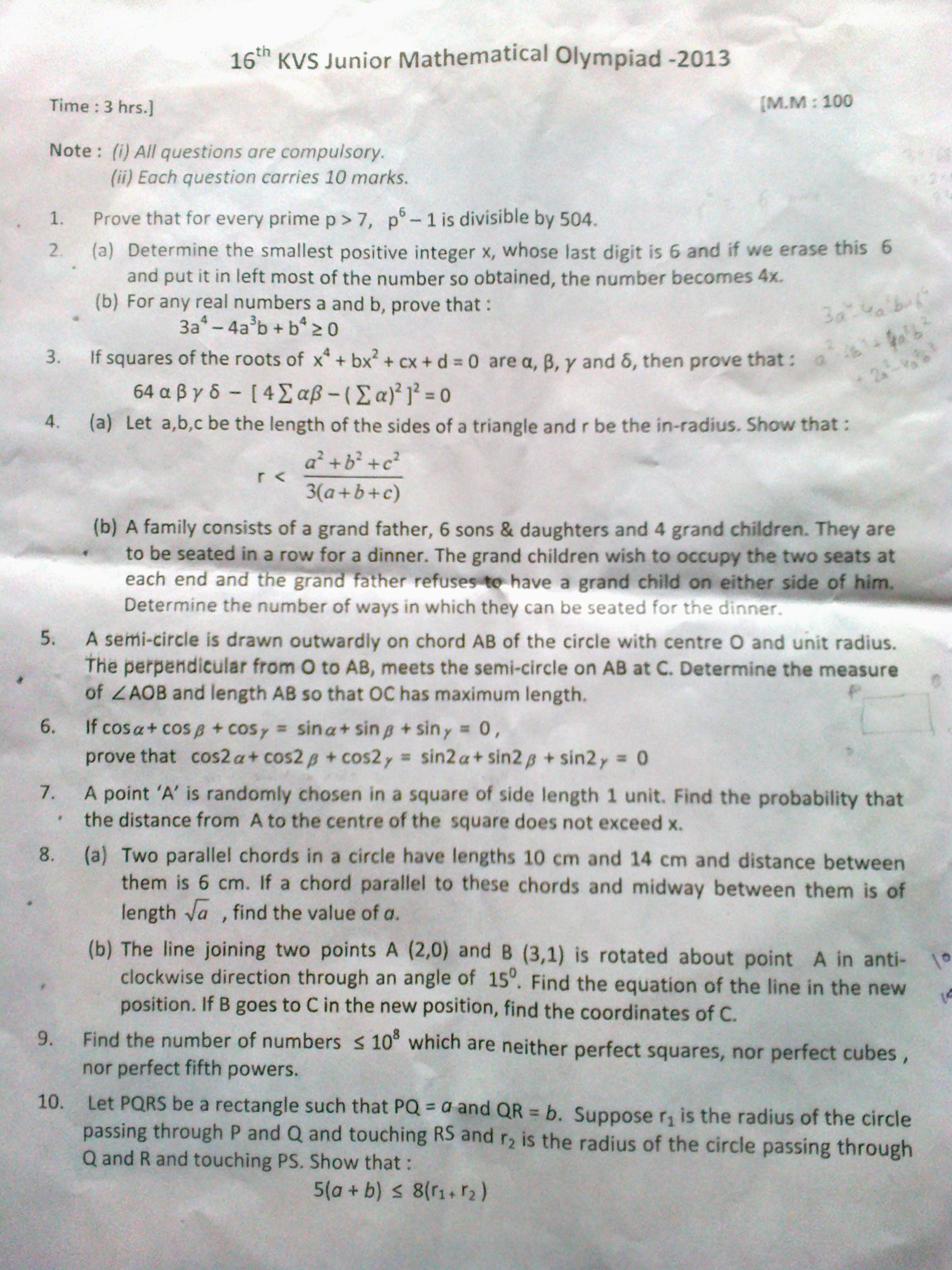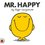Hello all, This is the question paper of JMO. Please help me with the solutions. Post solutions in the comment providing question number. Thanks.Note by Kumar Ashutosh
6 years, 9 months ago

This discussion board is a place to discuss our Daily Challenges and the math and science related to those challenges. Explanations are more than just a solution — they should explain the steps and thinking strategies that you used to obtain the solution. Comments should further the discussion of math and science.

When posting on Brilliant:

• Use the emojis to react to an explanation, whether you're congratulating a job well done , or just really confused .
• Ask specific questions about the challenge or the steps in somebody's explanation. Well-posed questions can add a lot to the discussion, but posting "I don't understand!" doesn't help anyone.
• Try to contribute something new to the discussion, whether it is an extension, generalization or other idea related to the challenge.

MarkdownAppears as
*italics* or _italics_ italics
**bold** or __bold__ bold
- bulleted- list
• bulleted
• list
1. numbered2. list
1. numbered
2. list
Note: you must add a full line of space before and after lists for them to show up correctly
paragraph 1paragraph 2

paragraph 1

paragraph 2

[example link](https://brilliant.org)example link
> This is a quote
This is a quote
    # I indented these lines
# 4 spaces, and now they show
# up as a code block.

print "hello world"
# I indented these lines
# 4 spaces, and now they show
# up as a code block.

print "hello world"
MathAppears as
Remember to wrap math in $$ ... $$ or $ ... $ to ensure proper formatting.
2 \times 3 $2 \times 3$
2^{34} $2^{34}$
a_{i-1} $a_{i-1}$
\frac{2}{3} $\frac{2}{3}$
\sqrt{2} $\sqrt{2}$
\sum_{i=1}^3 $\sum_{i=1}^3$
\sin \theta $\sin \theta$
\boxed{123} $\boxed{123}$

Sort by:

 Let $\cos \alpha + i\cdot \sin \alpha = e^{i\alpha}$

$\cos \beta + i\cdot \sin \beta = e^{i\beta}$

$\cos \gamma + i\cdot \sin \gamma = e^{i\gamma}$

Now Given $\cos \alpha + \cos \beta + \cos \gamma = 0 .........................(1)$

and $\sin \alpha + \sin \beta + \sin \gamma = 0.......................................(2) \times i$

$e^{i\alpha}+e^{i\beta}+e^{i\gamma} = 0...................................................(3)$

Now Square both Side

$e^{2i\alpha}+e^{2i\beta}+e^{2i\gamma} + 2 \left(e^{i(\alpha+\beta)}+e^{i(\beta+\gamma)}+e^{i(\gamma+\alpha)}\right) = 0$

Now in a $\triangle$ Sum of angle i.e $\alpha+\beta+\gamma = \pi\Leftrightarrow \alpha +\beta = \pi-\gamma$

So $e^{2i\alpha}+e^{2i\beta}+e^{2i\gamma} +e^{i(\pi-\gamma)}+e^{i(\pi-\beta)}+e^{i(\pi-\alpha)} = 0$

$e^{2i\alpha}+e^{2i\beta}+e^{2i\gamma} +e^{i(\pi-\gamma)}-2\left(e^{-i\alpha}+e^{-i\beta}+e^{-i\gamma}\right) = 0$

Now from $(3)$, Taking Congugate on both side, we get

$e^{-i\alpha}+e^{-i\beta}+e^{-i\gamma} = 0$

So $e^{2i\alpha}+e^{2i\beta}+e^{2i\gamma} = 0$

So $\cos 2\alpha + \cos 2\beta+\cos 2 \gamma+i\left(\sin 2 \alpha+\sin 2 \beta+\sin 2 \gamma\right) = 0+i\cdot 0$

we Get $\cos 2\alpha + \cos 2\beta+\cos 2 \gamma = 0$

and $\sin 2\alpha + \sin 2\beta+\sin 2 \gamma = 0$

- 6 years, 8 months ago

Question 10.

Firstly $r_1 = \tfrac{1}{8b}(a^2 + 4b^2)$ and $r_2 = \tfrac{1}{8a}(4a^2 + b^2)$. To see this, for example, suppose that $P$ has coordinates $(0,\tfrac12a)$, $Q$ coordinates $(0,-\tfrac12a)$. Then the centre of the first circle will have coordinates $(x,0)$ for some $x$, and the circle will touch $RS$ at its midpoint $(b,0)$. Then $x^2 + \tfrac14a^2 = (b-x)^2$, which gives us $x$. Then $r_1=b-x$. While $x$ could be negative, $r_1$ cannot.

Then $\begin{array}{rcl} r_1+r_2 & = & \frac{a(a^2+4b^2)+b(4a^2+b^2)}{8ab} \; = \; \frac{a^3+b^3 + 4a^2b + 4ab^2}{8ab} \\ & = & \frac{(a+b)(a^2-ab + b^2 + 4ab)}{8ab} \; = \; \frac{(a+b)(a^2 + 3ab + b^2)}{8ab} \\ & = & \tfrac{1}{8}(a+b)\big[3 + \tfrac{a}{b} + \tfrac{b}{a}\big] \; \ge \; \tfrac{1}{8}(a+b)[3+2] \; = \; \tfrac{5}{8}(a+b) \end{array}$

- 6 years, 9 months ago

- 6 years, 9 months ago

Did you try them already? Which did you manage to solve?

- 6 years, 9 months ago

I did q.1,2,4(b), 5,7,8, but try to give solutions of every question, so that I could verify my answers

- 6 years, 9 months ago

It would be better for you to state your solutions / approaches, and have others comment on it.

There can be subtleties in proof based questions, which would result in a 'similar-looking' answer being marked wrong.

Staff - 6 years, 9 months ago

Tell which one you couldn't solve .

- 6 years, 9 months ago

Please give solutions to all the questions you can, I will check the questions done by me and will understand what didn't solved.. Thanks

- 6 years, 9 months ago

Here are the anwers ( not for the proof - type questions.)

2(a) $\rightarrow 153846$

4(b) $\rightarrow$$7!4!\binom{6}{2}$

5 $\rightarrow$ 90

7 $\rightarrow \pi x^2$

8(a) $\rightarrow 184$

8(b) $\rightarrow x - y\sqrt{3} - 2 = 0$ , $(2 + \sqrt{\frac{3}{2}} , \frac{1}{\sqrt{2}})$

- 6 years, 9 months ago

yes, thanks, question 2(a), 5, 7, 8(a) matches with my solution, but can you give some explanation in 4(b). and I think your 8(b) is wrong. the angle of AC with x-axis is 60 degree. So, $\tan{60} = \frac {y - 0}{x - 2}$ Thus equation is $y= \sqrt{3}x - 2 \sqrt{3}$ which is my answer, and the coordinate also has some error. Am I right?

- 6 years, 9 months ago

ok , i went wrong in q.8(b) , it is rotated anti - clockwise , i rotated it clockwise.

4(b) Give end seats to grandchildren in $4\times3$ ways.

Now choose 1 seat out of 7 for grandfather( you can't select the seat which second from left or right.).in $7$ ways.

Then choose 2 seats out of 6 for remaining two grandchildren ( as we can't choose from grandfather's left or right and 3 seats are already occupied) and permutate in ${6 \choose 2}2!$ ways.

Now , six seats for sons and daughters remain , $6!$ ways for them.

Total no. of ways = $4\times3\times7\times{6 \choose 2}2!\times6! = 7!4!{6 \choose 2}$

- 6 years, 9 months ago

am I right in question 8(b), then what is the coordinate of C? In 4. how did you write- Give end seats to grandchildren in 4×3 ways? as far as I have understood the question,two seats each on the left and right ends of row is occupied by children. Am I wrong or You are mistaken..

- 6 years, 9 months ago

Left and Right end seats are occupied by grand children .

For left seat it has four options and right has three options ( as one is already taken by left one.). Hence by Fundamental principle of counting , no. of ways = $4\times 3$.

8(b) . $( 2 + \frac{1}{\sqrt{2}} , \sqrt{\frac{3}{2}})$

- 6 years, 9 months ago

okay, 8(b) is correct and also matches my solution.
In 4, I am still confused.Look and find error in my solution, it goes like this:

The extreme 2 seats on both ends are occupied by grand children. So 4 grand children occupy 4 seats in $4!$ ways. Numbering the seats 1,2,3.....11, we find that Grandfather canno sit on 3rd and 9th seat. So grandfather can sit on any one of 4th,5th,6th,7th,8th seats. This is done in $5$ ways.

Now after this arrangement, we have 6 seats left on which 6 son/daughter is to sit. This can be done in $6!$ ways

Therefore total possibilities= $4!.5.6!$

- 6 years, 9 months ago

- 6 years, 9 months ago

Challenge masters please give some hints!!!!!!!!!!

- 6 years, 9 months ago

The first question is very easy to solve. First of all, p^6 - 1 is divisible by 7, can be proved by fermat's little theorem. Next, p^6 -1 can be factorised to find whether it is divisible by 3^2, next the factorisation can also help to find whther it is divisble by 2^3. So 504 = 7* (3^2) * (2^3). This means p^6-1 is divisible by 504.

- 6 years, 9 months ago

Question 9 is nothing but inclusion-exclusion principle...perhaps!

- 6 years, 9 months ago

how to do it? if we can find the number of cubes less than $10^8$ and number of fifth power numbers less than $10^8$ then only we can solve it. Is there another way?

- 6 years, 9 months ago

Where and when is this exam held?

- 6 years, 9 months ago

is the answer of ques 8 34????

- 6 years, 9 months ago

which part, 8(a) or 8(b)

- 6 years, 9 months ago

(A)

- 6 years, 9 months ago

its 184

- 6 years, 9 months ago

2[b]:: Here $a,b\in \mathbb{R}$ and $3a^4-4a^3b+b^4\geq 0$

Now this is a Homogeneous equation, so Let $a = bx$ and Let $y = 3b^4x^4-4b^3x^3\cdot b+b^4$

So $y = b^4\cdot \left(3x^4-4x^3+1\right) = b^4\cdot (x-1)^2\cdot (3x^2+2x+1)\geq 0$

bcz $3x^2+2x+1 = 3\left(x+\frac{1}{3}\right)^2+\frac{8}{3}>0\;\forall x\in \mathbb{R}$

- 6 years, 8 months ago

There is also a simple answer to this. It goes like this:

We have, $3a^4-4a^3b+b^4$ $=a^4-2a^2b^2+b^4+2a^2b^2+2a^4-4a^3b$ $=(a^2-b^2)^2+2a^2(b^2+a^2-2ab)$ $= (a^2-b^2)^2+2a^2(a-b)^2 \geq 0$

- 6 years, 8 months ago